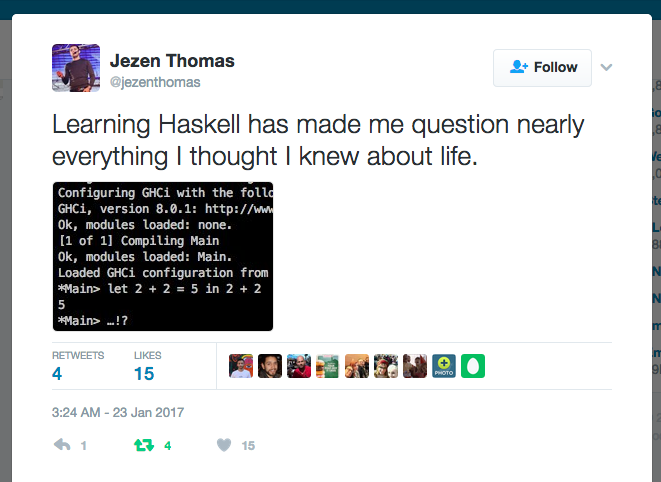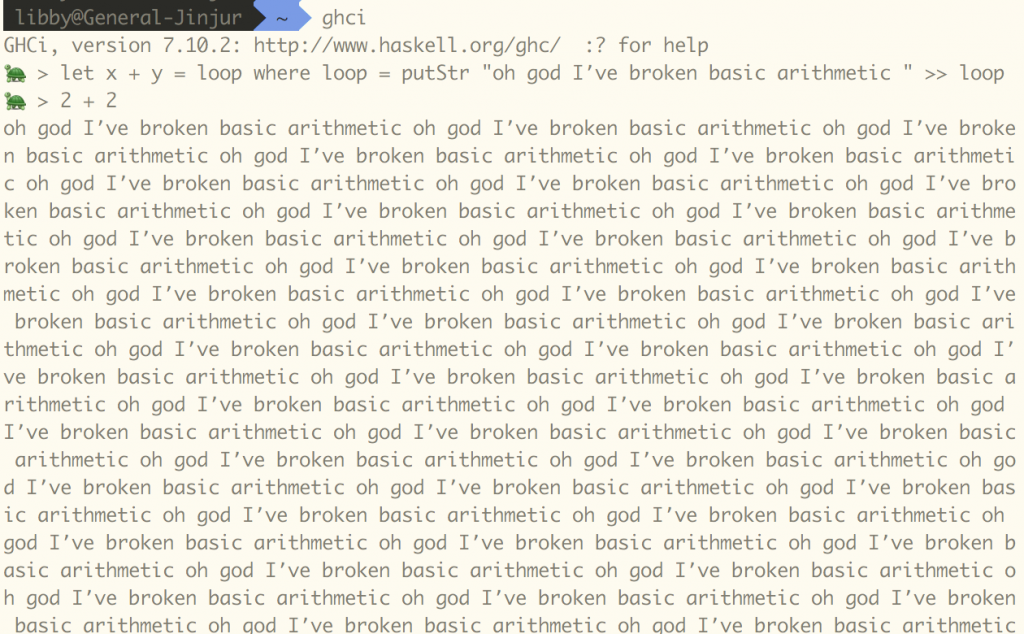2 + 2 = 5… !? and why compiler warnings are good

@jezenthomas posted this:(Twitter screencap) “Learning Haskell has made me question nearly everything I thought I knew about life.” (screencap of ghci session with the line `let 2 + 2 = 5 in 2 + 2` being evaluated to `5`.

To people who are very new to Haskell or who don’t know Haskell at all, the above probably looks really strange! Does two plus two equal five in Haskell? How? Why?

How to make 2 + 2 = 5

`let 2 + 2 = 5 in 2 + 2` is made up of two parts: `let 2 + 2 = 5 `and `in 2 + 2`. Let’s separate those out, because it turns out the weirdness still happens that way, and it’s also a little easier to understand.

Open up ghci, or go to ghc.io. Enter `2 + 2`. You probably won’t be too surprised that the answer is “4”.

But then type `let 2 + 2 = 5`. (ghci is totally fine with you making that nonsensical statement!)

Now try `2 + 2` again. This time the answer is 5.

What is going on here?

In ghci you can use the `let` keyword to define a named function or value. You could say `let hello name = "Hello, " ++ name`. This is a "total" function -- it’ll work with any String!

You can also define partial functions. A partial function is a function that only works with some of the possible inputs to that function.

You could change `hello` to be a partial function: `let hello "world" = "hello world"` takes one argument and it’ll throw an error if that argument is anything other than “world”.

`> let hello "world" = "hello world"> hello "friend"*** Exception: :2:5-33: Non-exhaustive patterns in function hello`

What does this error mean? A “pattern” is something that you’ve said that the function argument has to match. The pattern here is “world”. You’re giving it no other patterns that it can match. The function doesn’t know what to do with “friend”!

Partial functions make writing Haskell a lot harder than it should be, because they mean you can’t rely on the type of a function to tell you acceptable inputs. If a function takes a string as an argument, you should be able to give it any string, and not worry about your program just blowing up!

Is `let 2 + 2 = 5` a partial function? Let’s find out! Try `2 + 3` or `35 + 68` after you enter `let 2 + 2 = 5`. You’ll get an error like this:

`*** Exception: :2:5-13: Non-exhaustive patterns in function +`

In `2 + 2 = 5`, the pattern is that both arguments are “2”. You didn’t say what happens when the pattern is “2” and “3” or “35” and “68” -- or any other combination but “2” and “2”! You could change the pattern to match any input:

`let x + y = 5`

Now `2 + 2` still equals five, but so does `35 + 68` and `"apple" + "orange"`!

Why is this? If you haven’t guessed yet, the function that `let 2 + 2 = 5` is defining is the `(+)` function!

In some languages, operators like “+” and “-” are special. In Haskell, operators are just infix functions. (Most functions are “prefix” — you apply them by putting the name of the function first, then any arguments, like `hello "world"`. Infix functions can be used like operators, between the arguments, like `2 + 2` or `4 / 2`.)

It turns out that you can define `(+)` any way you like! You could define it like this:

`> let 2 + 2 = “apple”`

You could even make your function have some weird effect:

`> let x + y = loop where loop = putStr “oh god I’ve broken basic arithmetic ” >> loop`

(Watch out if you’re using ghc.io, this one breaks the “Safe Haskell” it uses!)

Now 2 + 2 = ….a ghci session with the looping declaration of 2 + 2 and and the repeated scrolling text “I’ve broken basic arithmetic”

So `(+)` is already defined by Haskell’s standard library as the ordinary addition operator, but you can call something else the same name. This is called “shadowing”.

Like partial functions, shadowing is usually a bad idea and often very confusing! You may think you are referring to one definition of some function or value when you’re actually referring to another. This can lead to very frustrating debugging sessions!

Why can 2 + 2 = 5? Can I make sure that 2 + 2 never equals 5?

It may seem really weird that you can just redefine a function like `(+)`. What happens if you just do that accidentally? Isn’t Haskell a language that’s supposed to be all pure and safe?

The good news is that you can avoid partial functions and shadowing in Haskell using compiler warnings. Type `:set -Wall` in ghci to set it to warn you for everything. (This doesn’t work in ghc.io.)

Then, if you try to write `2 + 2 = 5` you’ll get:

`:4:5: Warning:Pattern match(es) are non-exhaustiveIn an equation for ‘+’:Patterns not matched:#x _ with #x `notElem` [2#]2# #x with #x `notElem` [2#]`
`:4:7: Warning:This binding for ‘+’ shadows the existing bindingdefined at :2:7`

ghci is different from ghc

There is a difference between ghci (the interactive interpreter) and the way you would typically write Haskell when developing an application. In ghci, you’re writing a program line by line, but in typical development, you’d have some file that you’re writing code in and then compiling.

Make a file called “test.hs” and put this in it:

`2 + 2 = 5`
`main = print (2 + 2)`

Then try to compile it. You’ll get an error:

`\$ ghc test.hs[1 of 1] Compiling Main ( test.hs, test.o )`
`test.hs:1:17:Ambiguous occurrence ‘+’It could refer to either ‘Main.+’, defined at test.hs:3:3or ‘Prelude.+’,imported from ‘Prelude’ at test.hs:1:1(and originally defined in ‘GHC.Num’)`

The definition of `(+)` doesn’t get shadowed. Instead, there are two definitions of `(+)` and ghc doesn’t know which one you want. But you can still run into shadowing within functions. Which brings us back to this:

`main = let 2 + 2 = 5 in print (2 + 2)`
`\$ ghc test.hs[1 of 1] Compiling Main ( test.hs, test.o )Linking test ...\$ ./test5`

So yes, 2 + 2 can indeed equal 5 in Haskell. So make sure you turn on warnings!